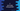# C program to sort array in ascending or descending order using pointer# Introduction :

In this C programming tutorial, we will learn how to sort elements of an array in ascending or descending order using C pointer. The program will take the array inputs from the user and sort the array in ascending or descending order.

## Pointer in C :

A variable is called a pointer variable in C if it can hold the address of a variable. Each pointer variable has specific data type e.g. an integer pointer variable can hold the address of an integer variable, a character pointer variable can hold the address of a character variable etc.

& is used to get the memory address of a variable. For example :

``int num = 10;``

For this integer variable, &num will give us the address of this variable. We can create one pointer variable to hold this address like below :

``int *p ;``

p can hold the address of an integer variable.

## Array and pointer :

We can store the address of an array in a pointer variable. The name of the array is the same as the address of the first element. We can store the address of the first element and access the other elements using an index.

For example, if int *p is a pointer to an array, its ith element can be accessed by *(p + i).

In this tutorial, we are using array pointers.

## C program :

``````#include <stdio.h>

//1
void swap(int *firstNumber, int *secondNumber);
void printArray(int *array, int size);

int main()
{
//2
int *array;
int type;
int i, j, size;

//3
printf("Enter the size of the array : ");
scanf("%d", &size);

//4
for (i = 0; i < size; i++)
{
printf("Enter element %d : ", (i + 1));
scanf("%d", (array + i));
}

//5
printf("Initial array : ");
printArray(array, size);

//6
printf("Enter 1 to sort in increasing order and 0 to sort in decreasing order : ");
scanf("%d", &type);

//7
for (i = 0; i < size; i++)
{
//8
for (j = i + 1; j < size; j++)
{
if (type == 1)
{
//increasing order sorting
if (*(array + j) < *(array + i))
{
swap((array + i), (array + j));
}
}
else
{
//decreasing order sorting
if (*(array + j) > *(array + i))
{
swap((array + i), (array + j));
}
}
}
}

//9
printf("Final array : ");
printArray(array, size);
return 0;
}

//10
void swap(int *firstNumber, int *secondNumber)
{
int temp = *firstNumber;
*firstNumber = *secondNumber;
*secondNumber = temp;
}

//11
void printArray(int *array, int size)
{
int i;
printf("[ ");
for (i = 0; i < size; i++)
{
printf("%d ", *(array + i));
}
printf("]\n");
}``````

## Explanation :

The commented numbers in the above program denote the step numbers below :

1. swap function is used to swap two numbers. It takes two integer pointers of two numbers and swaps them using the pointer. printArray is used to print an array. It takes one pointer to an array and the size of the array. Using the pointer, it prints its elements.
2. Create one pointer to an array and few variables.
3. Ask the user to enter the size of the array. Read the size and store it in the size variable.
4. Run one for loop to read the contents of the array. Read each element one by one.
5. Print the user entered array. We are using the printArray method to print the array elements.
6. Ask the user to enter the type of sorting. It reads the value and stores it in type variable. 1 is for ascending order and 0 is for descending order.
7. This is the main sorting part. For the first iteration of the loop, it is comparing the first element of the array to all other elements. Based on the sorting method, it finds out the largest or smallest element of the array. For the second iteration, it finds out the second largest or second smallest element etc. If you are getting confused with the loops, print all the values of i, j and other variables using printf. Try to analyze the printf logs and it will be clearer.
8. The inner loop is for comparing the elements right to the ith element with the ith element itself. Based on the value of type, it compares the element and swaps them both using swap function.
9. Finally, print the modified array to the user.
10. swap function takes two integer pointers and swaps the values lies in the address defined by these integers.
11. printArray function is used to print an array as mentioned above. It takes one pointer to an integer array and the length of the array.

### Sample Output :

``````Enter the size of the array : 5
Enter element 1 : 5
Enter element 2 : 4
Enter element 3 : 3
Enter element 4 : 2
Enter element 5 : 1
Initial array : [ 5 4 3 2 1 ]
Enter 1 to sort in increasing order and 0 to sort in decreasing order : 1
Final array : [ 1 2 3 4 5 ]

Enter the size of the array : 5
Enter element 1 : 3
Enter element 2 : 8
Enter element 3 : 23
Enter element 4 : 123
Enter element 5 : 9
Initial array : [ 3 8 23 123 9 ]
Enter 1 to sort in increasing order and 0 to sort in decreasing order : 0
Final array : [ 123 23 9 8 3 ]``````

## Conclusion :

In this C tutorial, we have learned how to sort the elements of an array in ascending or descending order using C pointer. Try to run the program and drop one comment below if you have any queries.